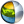Cg 3.1 Toolkit Documentation Cg / Standard Library / determinant

## Name

determinant - return the scalar determinant of a square matrix

## Synopsis

```float determinant(float1x1 A);
float determinant(float2x2 A);
float determinant(float3x3 A);
float determinant(float4x4 A);
```

## Parameters

A
Square matrix of which to compute the determinant.

## Description

Returns the determinant of the square matrix A.

## Reference Implementation

The various determinant functions can be implemented like this:

```float determinant(float1x1 A)
{
return A._m00;
}

float determinant(float2x2 A)
{
return A._m00*A._m11 - A._m01*A._m10;
}

float determinant(float3x3 A)
{
return dot(A._m00_m01_m02,
A._m11_m12_m10 * A._m22_m20_m21
- A._m12_m10_m11 * A._m21_m22_m20);
}

float determinant(float4x4 A) {
return dot(float4(1,-1,1,-1) * A._m00_m01_m02_m03,
A._m11_m12_m13_m10*(  A._m22_m23_m20_m21*A._m33_m30_m31_m32
- A._m23_m20_m21_m22*A._m32_m33_m30_m31)
+ A._m12_m13_m10_m11*(  A._m23_m20_m21_m22*A._m31_m32_m33_m30
- A._m21_m22_m23_m20*A._m33_m30_m31_m32)
+ A._m13_m10_m11_m12*(  A._m21_m22_m23_m20*A._m32_m33_m30_m31
- A._m22_m23_m20_m21*A._m31_m32_m33_m30));
}
```

## Profile Support

determinant is supported in all profiles except fp20, vs_1_1, ps_1_1, ps_1_2 and ps_1_3. However profiles such as ps_2_0 without native floating-point will have problems computing the larger determinants and may have ranges issues computing even small determinants.| Cg Toolkit | Download | Release Archive | Profiles | Reference | Books | Discussions |